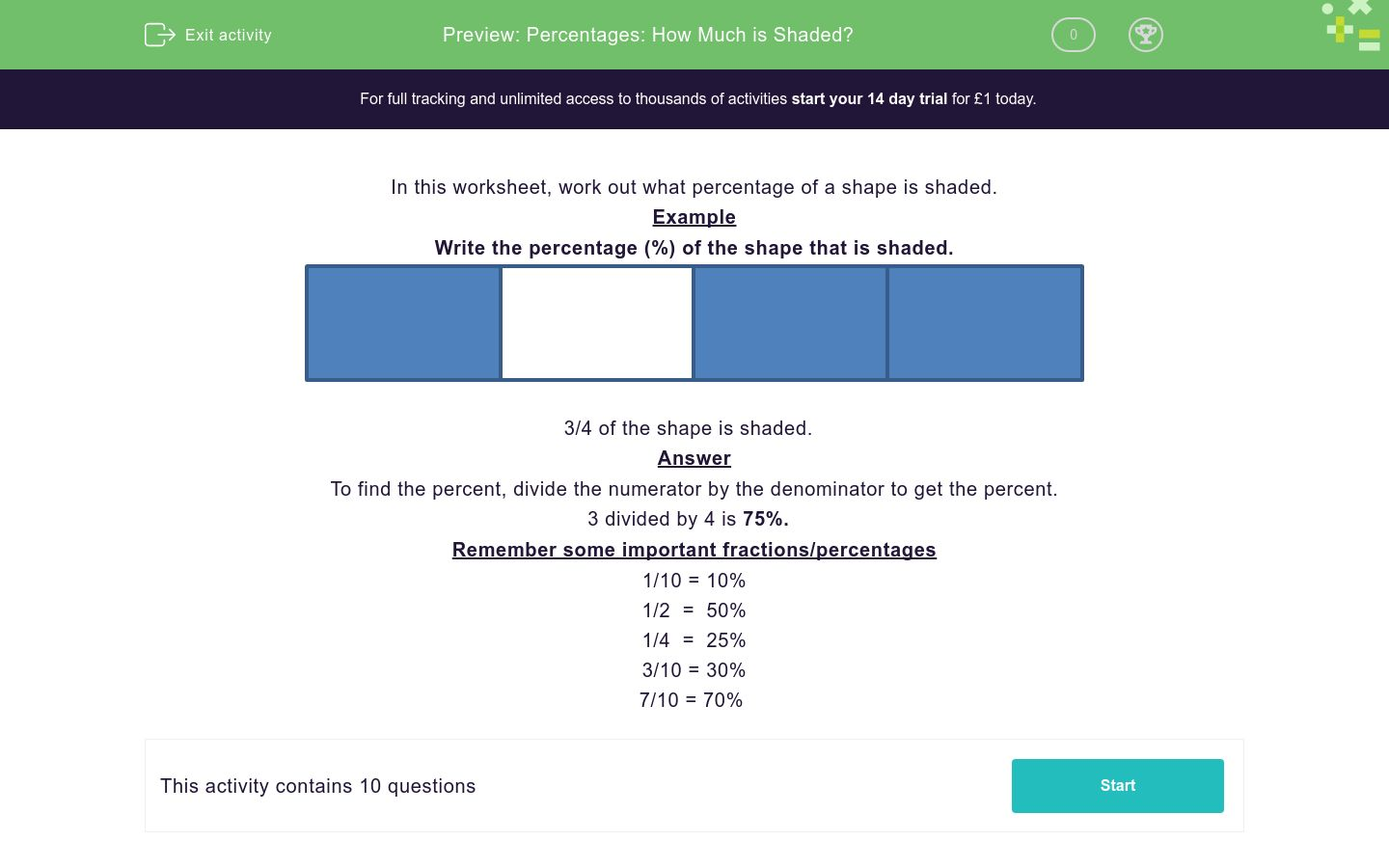# Percentages: How Much is Shaded?

In this worksheet, students use what they know about percentages to work out what percentage of a shape is shaded.Key stage:  KS 2

Curriculum topic:  Ratio and Proportion

Curriculum subtopic:  Solve Percentage Problems

Difficulty level:### QUESTION 1 of 10

In this worksheet, children must work out what percentage of a shape is shaded.

Example

Write the percentage (%) of the shape that is shaded.8 tenths of the shape is shaded.  This is 80%.  (Just write 80 in the answer box)

Remember some important fractions/percentages

1/10 = 10%

3/10 = 30%

7/10 = 70% etc..

1/2  =  50%

1/4  =  25%

Write the percentage (%) of the shape that is shaded. Don't include the percent symbol.Write the percentage (%) of the shape that is shaded. Don't include the percent symbol.Write the percentage (%) of the shape that is shaded. Don't include the percent symbol.Write the percentage (%) of the shape that is shaded. Don't include the percent symbol.Write the percentage (%) of the shape that is shaded. Don't include the percent symbol.Write the percentage (%) of the shape that is shaded. Don't include the percent symbol.• Question 1

Write the percentage (%) of the shape that is shaded. Don't include the percent symbol.100
• Question 2

Write the percentage (%) of the shape that is shaded. Don't include the percent symbol.80
• Question 3

Write the percentage (%) of the shape that is shaded. Don't include the percent symbol.25
• Question 4

Write the percentage (%) of the shape that is shaded. Don't include the percent symbol.50
• Question 5

Write the percentage (%) of the shape that is shaded. Don't include the percent symbol.50
• Question 6

Write the percentage (%) of the shape that is shaded. Don't include the percent symbol.